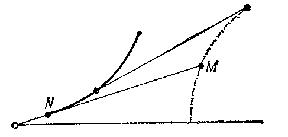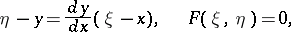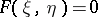# Curve of pursuit

(diff) ← Older revision | Latest revision (diff) | Newer revision → (diff)

A curve representing a solution of the "pursuit" problem, which is posed as follows: Let a pointbe moved uniformly along a given curve. The trajectory has to be found of the uniform movement of a point, such that the tangent drawn towards the trajectory at any moment of the movement would pass through the position ofcorresponding to that moment in time.Figure: c027400a

In a plane, the system of equations which the curve of pursuit must satisfy takes the formwhereis the slope of the curve of pursuit, andis the equation of the given curve.

The "pursuit" problem was posed by Leonardo da Vinci and solved by P. Bouguer (1732). For a generalization of it see the last Chapter of .

How to Cite This Entry:
Curve of pursuit. Encyclopedia of Mathematics. URL: http://encyclopediaofmath.org/index.php?title=Curve_of_pursuit&oldid=15061
This article was adapted from an original article by D.D. Sokolov (originator), which appeared in Encyclopedia of Mathematics - ISBN 1402006098. See original article